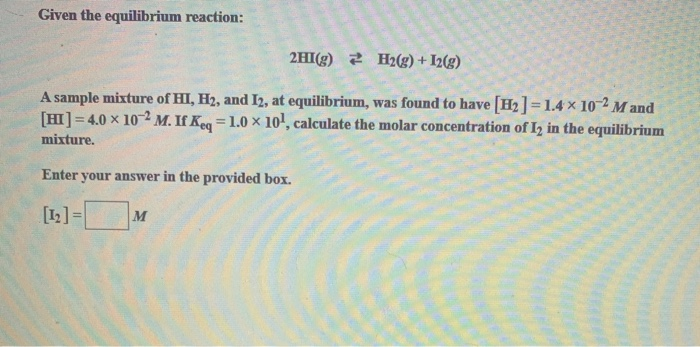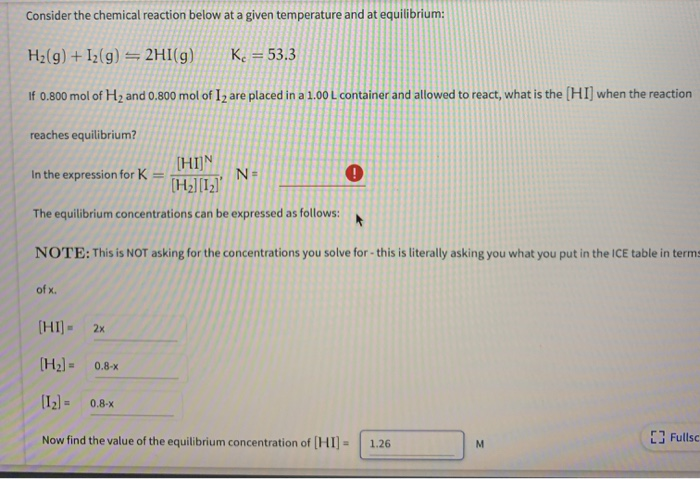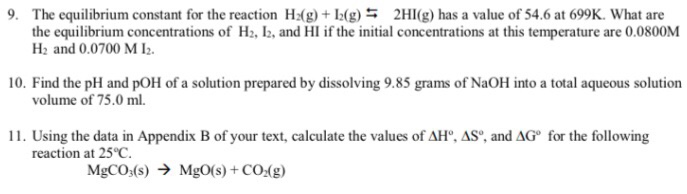Question

# Consider the following chemical reaction: H2 (g) + I2 (g) 2HI (g) At equilibrium in a...

Consider the following chemical reaction: H2 (g) + I2 (g) 2HI (g) At equilibrium in a particular experiment, the concentrations of H2, I2, and HI were 0.02, 0.021 and 0.160 M respectively. The value of Keq for this reaction is __________.

We need at least 10 more requests to produce the answer.

0 / 10 have requested this problem solution

The more requests, the faster the answer.

All students who have requested the answer will be notified once they are available.

#### Earn Coins

Coins can be redeemed for fabulous gifts.

Similar Homework Help Questions
• ### Given that the reaction H2 (g) + I2 (g) <--> 2HI (g) is at equilibrium and...

Given that the reaction H2 (g) + I2 (g) <--> 2HI (g) is at equilibrium and the measured concentrations of each component of the reaction are [H2] = 0.22 M, [I2] = 0.22 M, and [HI] = 1.56 M, write the equilibrium expression and then calculate Keq.

• ### Given the equilibrium reaction: 2HI(g) H2(g) + I2(g) A sample mixture of HI, H2, and 12,...Given the equilibrium reaction: 2HI(g) H2(g) + I2(g) A sample mixture of HI, H2, and 12, at equilibrium, was found to have [H2]- 1.4 x 102 Mand [HI 4.0 x 102 M. If Keq 1.0 x 10, calculate the molar concentration of I2 in the equilibrium mixture, Enter your answer in the provided box. ]= м

• ### The value of Keq for the equilibrium H2 (g) + I2 (g) ⇌ 2HI (g) is...

The value of Keq for the equilibrium H2 (g) + I2 (g) ⇌ 2HI (g) is 794 at 25 °C. At this temperature, what is the value of Keq for the equilibrium below? HI (g) ⇌ 1/2 H2 (g) + 1/2 I2 (g) 1588 0.0013 397 28 0.035

• ### Consider the chemical reaction below at a given temperature and at equilibrium: H2(g) +12(g) = 2HI(g)...Consider the chemical reaction below at a given temperature and at equilibrium: H2(g) +12(g) = 2HI(g) Kc = 53.3 If 0.800 mol of H2 and 0.800 mol of 12 are placed in a 1.00L container and allowed to react, what is the [HI] when the reaction reaches equilibrium? [HIN In the expression for K N- (H2] ' The equilibrium concentrations can be expressed as follows: NOTE: This is NOT asking for the concentrations you solve for this is literally asking...

• ### For the reaction in the previous problem, that is, 2HI(g) ↔ H2(g) + I2(g) Keq =...

For the reaction in the previous problem, that is, 2HI(g) ↔ H2(g) + I2(g) Keq = 0.016 Initially a container contains 0.39 M HI and no product. What is the equilibrium concentration of H2?

• ### The equilibrium constant for the reaction: H2(g) + I2(g) <--> 2HI(g) is 54 at 700 K....

The equilibrium constant for the reaction: H2(g) + I2(g) <--> 2HI(g) is 54 at 700 K. A mixture of H2, I2 and HI, each at 0.020 M, was introduced into a container at 700 K. Which of the following is true? At equilibrium, [H2] = [I2] = [HI]. No net change occurs because the system is at equilibrium. The reaction proceeds to the left producing more H2(g) and I2(g). The reaction proceeds to the right producing more HI(g). At equilibrium,...

• ### For the reaction: 2HI(g) ↔ H2(g) + I2(g) Keq = 0.016 Initially a container contains 0.60...

For the reaction: 2HI(g) ↔ H2(g) + I2(g) Keq = 0.016 Initially a container contains 0.60 M HI, 0.038 M H2, and 0.15 M I2 at equilibrium. What is the new equilibrium concentration of H2, if the H2 concentration is increased by 0.276 M?

• ### 9. The equilibrium constant for the reaction H2(g) + I2(g) 2HI(g) has a value of 54.6 at 699K. ...9. The equilibrium constant for the reaction H2(g) + I2(g) 2HI(g) has a value of 54.6 at 699K. What are the equilibrium concentrations of H2, I2, and HI if the initial concentrations at this temperature are 0.0800M H2 and 0.0700 M I2. 10. Find the pH and pOH of a solution prepared by dissolving 9.85 grams of NaOH into a total aqueous solution volume of 75.0 ml. 11. Using the data in Appendix B of your text, calculate the values...

• ### Consider the equilibrium reaction. H2(g) + I2(g) equilibrium reaction arrow 2 HI(g) In this case, 1.000...

Consider the equilibrium reaction. H2(g) + I2(g) equilibrium reaction arrow 2 HI(g) In this case, 1.000 M H2 reacts with 2.000 M of I2 at a temperature of 414°C. The value of Kc = 72. Determine the equilibrium concentrations of H2, I2, and HI. [H2] [I2] [HI]

• ### The equilibrium constant, Kc, for the following reaction is 55.6 at 698 K: H2(g) + I2(g)...

The equilibrium constant, Kc, for the following reaction is 55.6 at 698 K: H2(g) + I2(g) 2HI(g) Calculate the equilibrium concentrations of reactants and product when 0.293 moles of H2 and 0.293 moles of I2 are introduced into a 1.00 L vessel at 698 K. [H2] = M [I2] = M [HI] = M